# C++ STL常用标准库容器入门（vector,map,set,string,list...）

STL常用标准库容器

C++ STL中最基本以及最常用的类或容器无非就是以下几个：

# string

char* s1 = "Hello JackYu!"; //创建指针指向字符串常量，既然是常量字符串，这段字符串我们是不能修改的

//想要创建 可以修改的字符串，我们可以使用数组分配空间
char s2 = "Hello JackYu!";
//或者这样
char s3[] = "Hello JackYu!";

//当然我们也可以手动的动态分配内存
char* s4 = (char*)malloc(20）;
gets(s4);

C++ 标准库中的string表示可变长的字符串，它在头文件string里面。

#include <string>
using std::string;

### 直接初始化和拷贝初始化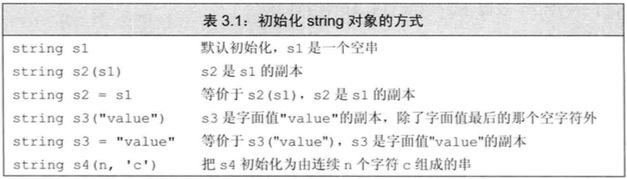string s1;//初始化字符串，空字符串
string s2 = s1; //拷贝初始化，深拷贝字符串
string s3 = "I am b"; //直接初始化，s3存了字符串
string s4(10, 'a'); //s4存的字符串是aaaaaaaaaa
string s5(s4); //拷贝初始化，深拷贝字符串
string s6("I am d"); //直接初始化
string s7 = string(6, 'c'); //拷贝初始化，cccccc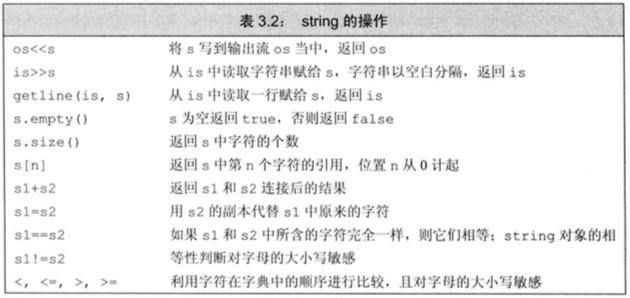//
//  main.cpp
//  LearnEffective C++
//
//  Created by 于磊 on 2018/6/24.
//

#include <iostream>
using std::string;
using std::cout;
using std::endl;
using std::cin;
int main(int argc, const char * argv[]) {
string s1;//初始化字符串，空字符串
string s2 = s1; //拷贝初始化，深拷贝字符串
string s3 = "Hellor World"; //直接初始化，s3存了字符串
string s4(10, 'a'); //s4存的字符串是aaaaaaaaaa
string s5(s4); //拷贝初始化，深拷贝字符串
string s6("Scott"); //直接初始化
string s7 = string(6, 'c'); //拷贝初始化，cccccc

//string的各种操作
string s8 = s3 + s6;//将两个字符串合并成一个
s3 = s6;//用一个字符串来替代另一个字符串的对用元素

cin >> s1;

cout << s1 << endl;
cout << s2 << endl;
cout << s3 << endl;
cout << s4 << endl;
cout << s5 << endl;
cout << s6 << endl;
cout << s7 << endl;
cout << s8 << endl;
cout << "s7 size = " << s7.size() << endl; //字符串长度，不包括结束符
cout << (s2.empty() ? "This is empty string" : "This string is not empty") << endl;;

return 0;
}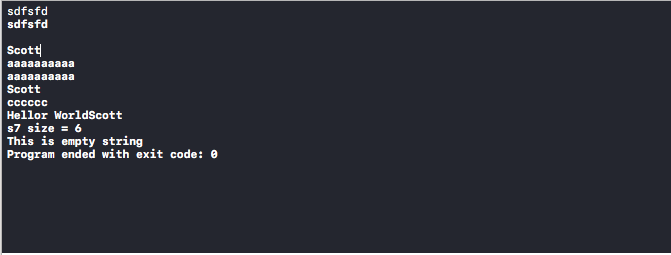### string的IO操作

"     Hello   World"

cin>>s1>>s2;

hello存在s1里，world存在s2里了。

string str;
getline(cin, str);
cout << str << endl;

string s1 = s2 + ", "; //正确,此处其实包含了隐式的类型转换,string对象+字符串常量转成了string对象
string s3 = "s " + ", "; //错误,const char *中没有此算术运算符
string s4 = "hello" + ", " + s1; //错误,同上
string s5 = s1 + "hello " + ", "; //改一下顺序，s1放前头，正确了，注意理解=号右边的运算顺序

 for(auto &iter:s6){
cout<<iter<<endl;
iter = '1';
}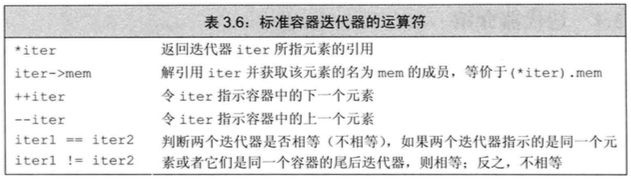string str1("some string");
if (str1.begin() != str1.end()) {
auto it = str1.begin();
*it = toupper(*it);
}
cout << str1 << endl;
string str2("some string");//yl
for (string::iterator it = str2.begin(); it != str2.end(); it++) {
*it = toupper(*it);
}
cout << str2 << endl;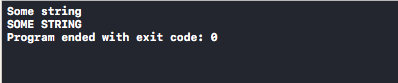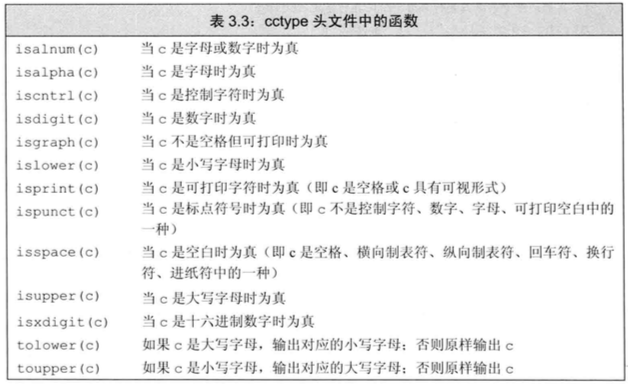string还有一些很好用的算法，比如找子串

string sq("aaaaa bbb cc s");
cout << sq.find("aa", 0) << endl; //返回的是子串位置。yl第二个参数是查找的起始位置，如果找不到，就返回string::npos
if (sq.find("aa1", 0) == string::npos)
{
cout << "找不到该子串！" << endl;
}

### string中包含的专有的操作（相对于vector来说）

1.  string的添加与替换

在string中，增加了append(）与 replace()函数

str.append(args)　　　　// 在尾部添加一个字符或一个字符

str.replace(pos, args)　　　　// 在尾部添加一个字符或一个字符 ,它的重载函数很多,共16个。

2. string的访问子字符串：

str.substr(_pos, n)　　//该函数可以获得原字符串中的部分字符, 从pos开始的n个字符，当_pos超过范围时，会抛出out_of_range的异常yl。

3. str的搜索操作：

str.find(args)　　//查找args 第一次出现的位置

str.rfind(args)　　//查找args最后一次出现的位置

str.find_first_of(args)　　　//搜索的是字符， 第一个是args里的字符的位置y

str.find_last_of(args)　　　// 搜索的是字符， 最后一个是args里的字符的位置l

str.find_first_not_of()　　// 搜索的是字符，第一个不是args里的字符的位置

str.find_last_not_of()　　// 搜索的是字符， 最后一个不是args里的字符的位置

4. str的大小操作：

str.length()　　　// 该函数与str.size()函数完成一样，只是名字不同而已罢了。只所以这样搞的原因，可能开发人员感觉length更适合字串符，size更适合容器吧。

### c字符串的转换函数

1. 由数值转换为字符串：

to_string(val): 

2. 由字符串转换为数值：（要转换的string的第一个非空白符必须是数值中可能出现的字符，处理直到不可能转换为数值的字符为止，以下内容来自：c++primer）

stoi(str, pos, base)  　　// 字符串转换为整型，其中str表示字符串，  pos用于表示第一个非数值字符的下标(意思就是我给函数传入一个地址，它会对它进行赋第一个非数值字符的位置）, base表数值的基数，默认为10，即10进制数。yulei
stol(str, pos, base)　　　　// 转换为long
stoul(str, pos, base)　　　　// 转换为 unsigned long
stoll(str, pos, base)　　　　// 转换为 long long
stoull(str, pos, base)　　　// 转换为unsigned long long
stof(str, pos)　　　　　　// 转换为float
stod(str, pos,)　　　　　// 转换为double
stold(str, pos,)　　　　　// 转换为long double

# vector

vector是数组的一种类表示，它提供了自动内存管理功能，可以动态地改变vector对象的长度，并随着元素的添加和删除而增大缩小，

vector存在于头文件vector中

#include <vector>

### 初始化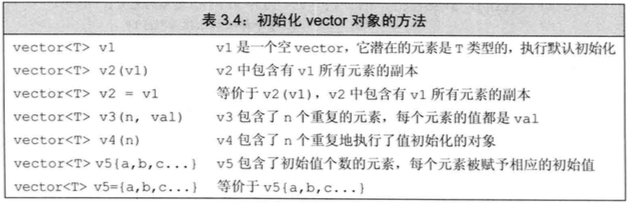vector<int> v1;
vector<father> v2;
vector<string> v3;
vector<vector<int> >;  //注意空格。这里相当于二维数组int a[n][n]嵌套vector;
vector<int> v5 = { 1,2,3,4,5 }; //C11列表初始化
vector<string> v6 = { "hi","C++","Primer","learning","hard yl" };
vector<int> v7(5, -1); //初始化为-1,-1,-1,-1,-1。第一个参数是数目，第二个参数是要初始化的值
vector<string> v8(3, "hi");
vector<int> v9(10); //默认初始化为0
vector<string> v10(4); //默认初始化为空字符串

for (int i = 0; i < 5; i++)
{
vec.push_back(i);
}

vector其他成员函数操作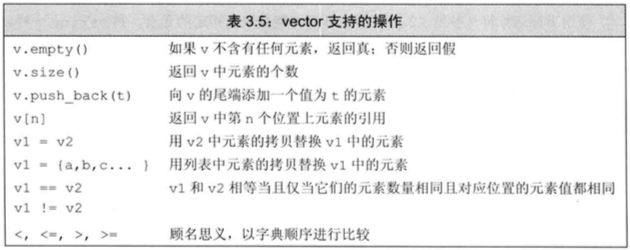for (int i = 0; i < vec.size(); i++)
{
cout << vec[i] << endl;
vec[i] = 100;              //不建议这样写数据
cout << vec[i] << endl;
}

vector<int> vec;
vec = 1;  //错误！

vector<string> v6 = { "hi","C++","Primer","learning","hard" };
for (vector<string>::iterator iter = v6.begin(); iter != v6.end(); iter++)
{
cout << *iter << endl;
//下面两种方法都行,对象和引用的区别
cout << (*iter).empty() << endl;
cout << iter->empty() << endl;
}
//反向迭代器
for (vector<string>::reverse_iterator iter = v6.rbegin(); iter != v6.rend(); iter++)
{
cout << *iter << endl;

}

vector最常用的增删操作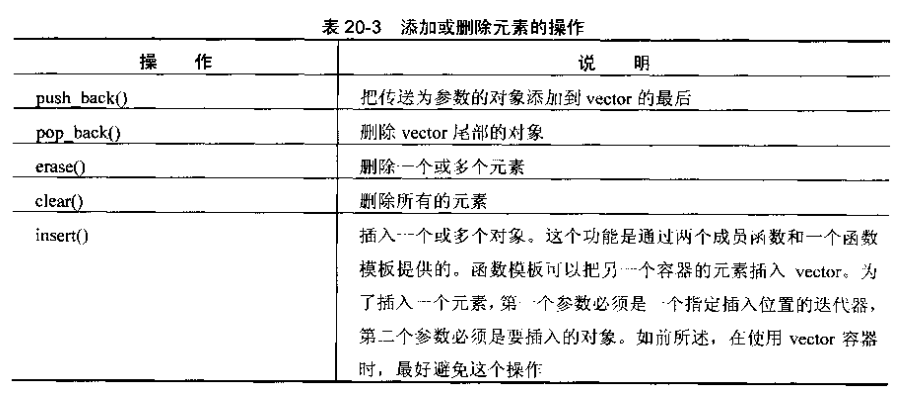//
//  main.cpp
//  LearnEffective C++
//
//  Created by 于磊 on 2018/6/24.
//

#include <iostream>
#include <vector>
#include <string>
using namespace std;

template <typename T> void showvector(vector<T> v) {
for (typename vector<T>::iterator it = v.begin(); it != v.end(); it++) {
cout << *it;
}
cout << endl;
}

int main(int argc, const char *argv[]) {
vector<string> v6 = {"hi", "C++", "Primer", "learning", "hard"};
v6.resize(3); //重新调整vector容量大小
showvector(v6);

vector<int> v5 = {1, 2, 3, 4, 5}; //列表初始化,注意使用的是花括号
cout << v5.front() << endl;       //访问第一个元素
cout << v5.back() << endl;        //访问最后一个元素

showvector(v5);
v5.pop_back(); //删除最后一个元素
showvector(v5);
v5.push_back(6); //加入一个元素并把它放在最后
showvector(v5);
v5.insert(v5.begin() + 1, 9); //在第二个位置插入新元素
showvector(v5);
v5.erase(v5.begin() + 3); //删除第四个元素
showvector(v5);
v5.insert(v5.begin() + 1, 7,8); //连续插入7个8
showvector(v5);
v5.clear(); //清除所有内容
showvector(v5);

return 0;
}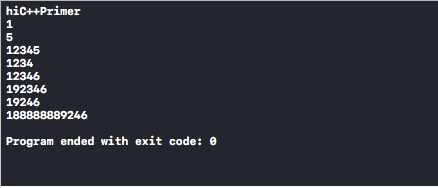### vecotr常使用的操作

1. 属性操作

v1.size()　　　　　　//v1内已经存放的元素的数目
v1.capacity()　　　　// v1现有的在存储容量（不再一次进行扩张内存空间的前提下）
v1.empty()　　　　　// 判断v1是否为空
v1.max_size()　　　 // 返回vector可以存放的最大元素个数，一般这个数很大，因为vector可以不断调整容量大小。
v1.shrink_to_fit()　　// 该函数会把v1的capacity()的大小压缩到size()大小，即释放多余的内存空间。

2. 访问操作：访问操作都会返回引用，通过它，我们可以修改vector中的值。

v1[n]　　　　　　　　// 通过下标进行访问vector中的元素的引用 （下标一定要存在 ，否则未定义，软件直接崩了）
v1.at(n)　　　　　　　// 与上面类似，返回下标为n的元素的引用，不同的是，如果下标不存在，它会抛出out_of_range的异常。它是安全的，建议使用它。
v1.front()　　　　　　// 返回vector中头部的元素的引用（使用时，一定要进行非空判断）
v1.back()　　　　　　// 返回vector中尾部的元素 引用（使用时，一定要进行非空判断）

3. 添加操作：

v1.push_back(a)　　　　　　　　//在迭代器的尾部添加一个元素
v1.push_front(a)　　　　　　　　// vector不支持这个操作
v1.insert(iter,  a)　　　　　　　　// 将元素a 插入到迭代器指定的位置的前面，返回新插入元素的迭代器（在c++11标准之前的版本，返回void)
v1.insert(iter, iter1, iter2)　　　    //把迭代器[iterator1, iterator2]对应的元素插入到迭代器iterator之前的位置，返回新插入的第一个元素的迭代器（在c++11标准之前的版本， 返回空）。

在c++11标准中，引入了emplac_front()、 emplace()、emplace_back(), 它们分别与push_front()、insert()、 push_back()相对应，用法与完成的动作作完全相同，但是实现不一样。 push_front()、insert()各push_back()是对元素使用copy操作来完成的，而emplac_front()、 emplace()和emplace_back()是对元素使用构造来完成的，后者的效率更高，避免了不必要的操作。因此，在以后更后推荐使用它们。

4. 删除操作：

v1.erase(iterator)　　　　　// 删除人人迭代器指定的元素，返回被删除元素之后的元素的迭代器。（效率很低，最好别用）
v1.pop_front() 　　　　　　//vector不支持这个操作
v1.pop_back()　　　　　　//删除vector尾部的元素 ， 返回void类型 (使用前，一定要记得非空判断）
v1.clear()　　　　　　　　 //清空所有元素

5. 替换操作：

v1.assign({初始化列表})　　　　// 它相当于赋值操作，
v1.assign(n, T)　　　　　　　　// 此操作与初始化时的操作类似，用个n T类型的元素对v1进行赋值
v1.assign(iter1, iter2)　　　　　// 使用迭代器[iter1, iter2]区间内的元素进行赋值（该迭代器别指向自身就可以），另外，只要迭代器指的元素类型相同即可（存放元素的容器不同，例如：可以用list容器内的值对vector容器进行assign操作，而用 "=" 绝对做不到的。
v1.swap(v2)　　　　　　// 交换v1与v2中的元素。 

swap操作速度很快，因为它是通过改变v1与v2两个容器内的数据结构（可能是类似指针之类的与v1和v2的绑定）完成的，不会对容器内的每一个元素进行交换。 这样做，不仅速度快，并且指向原容器的迭代器、引用以及指针等仍然有效，因为原始的数据没有变。在c++ primer 中建议大家使用非成员版本的swap()函数，它在范型编程中很重要。

# deque

deque模版类 double-ended queue(双端队列) ，支持随机访问，从deque对象的开始位置插入和删除元素的时间是固定的，而不像vector是线性时间。

# list

list（双向链表），除了第一个和最后一个元素外，每个元素都与前后的元素相连接，这意味着可以双向遍历链表，list和vector之间关键区别在于，list在链表中任一位置进行插入和删除的时间都是固定的（vector模版提供了除结尾处外的线性时间的插入和删除，在结尾处，它提供了固定时间的插入和删除）。因此，vector强调的是通过随机访问快速访问，而list强调的是元素的快速插入和删除。list也可以反转容器，list不支持数组表示法和随机访问。因为它是用双向链表实现的，所以，它的一大特性就是它的迭代器永远不会变为无效（除非这段空间不存在了），即无论增加、删除操作，都不会破坏迭代器。list是一个双向链表，而单链表对应的容器则是foward_list。

list的头文件

#include <list>

//
//  main.cpp
//  set
//
//  Created by yulei on 2018/8/10.
//

#include <iostream>
#include <list>
#include <string>
using namespace std;

template <typename T> void showlist(list<T> v) {
for (typename list<T>::iterator it = v.begin(); it != v.end(); it++) {
cout <<" "<< *it;
}
cout << endl;
}
int main(int argc, const char *argv[]) {

list<int> lst1{1, 2, 3, 4, 5, 6, 7};
showlist(lst1);
list<double> l2;
list<char> lst3(10);
list<int> lst2(5, 10); //将元素都初始化为10
showlist(lst2);
return 0;
}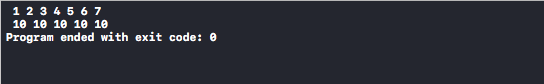list<int> lst1{1, 4, 343, 5, 666, 2, 3, 4, 5, 6, 7};
showlist(lst1);
lst1.sort();
showlist(lst1);

# forward_list

forward_list，它实现了单链表，在这种链表中，每个节点都只链接到下一个节点，而没有链接到前一个节点。因此forward_list只需要正向迭代器，而不需要双向迭代器。因此，不同于vector和list，forward_list是不可反转的容器。相比list，forward_list更简单，更紧凑，但功能也更少。

# queue

queue，queue模版的限制比deque更多，它不仅不允许随机访问队列元素，甚至不允许遍历队列。它把使用限制在定义队列的基本操作上，可以将元素添加到队尾，从队首删除元素，查看队首和队尾的值，检查元素数目和测试队列是否为空。

# priority_queue

priority_queue，和queue的区别在于，最大的元素被移到队首。内部区别在于，默认的底层类是vector。可以修改用于确定哪个元素放到队首的比较方式。方法是提供一个构造函数。

# stack

7.stack,提供了典型的栈接口,stack模版的限制比vector更多。它不仅不允许随机访问栈元素，甚至不允许遍历栈，它把使用限制在定义栈的基本操作上，即可以将压入推到栈顶，从栈顶弹出元素，查看栈顶的值，检查元素数目和测试栈是否为空.于queue想死，如果要使用栈中的值，必须首先使用top()来检索这个值，然后使用pop将它中栈中删除。

# set

### 头文件

#include <set>
//
//  main.cpp
//  set
//
//  Created by yulei on 2018/8/10.
//

#include <iostream>
#include <string>
#include <set>
using namespace std;

template <typename T> void showset(set<T> v) {
for (typename set<T>::iterator it = v.begin(); it != v.end(); it++) {
cout << *it << " ";
}
cout << endl;
}
int main(int argc, const char *argv[]) {

set<int> s1{91, 8, 341, 3, 3, 4, 3, 3, 4, 5, 66, 6, 1, 5, 5, 6, 7, 7}; //自动排序，从小到大,剔除相同项
showset(s1);
set<string> s2{"hello", "C++", "Primer", "5th"}; //字典序排序
showset(s2);
s1.insert(9); //有这个值了，do nothing
showset(s1);
s2.insert("aaa"); //没有这个字符串，添加并且排序
showset(s2);

return 0;
}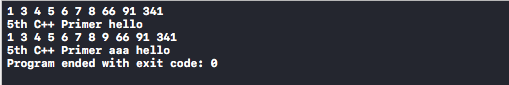# map

map运用了哈希表地址映射的思想，也就是key-value的思想，来实现的，底层和set一样都是用红黑树实现。内部元素按照key哈希排序。首先给出map最好用也最最常用的用法例子，就是用字符串作为key去查询操作对应的value。

### 头文件

#include <map>
//
//  main.cpp
//  set
//
//  Created by yulei on 2018/8/10.
//

#include <iostream>
#include <string>
#include <map>
using namespace std;

void showmap(map<string, int> v)
{
for (map<string, int>::iterator it = v.begin(); it != v.end(); it++)
{
cout << it->first << "  " << it->second << endl;  //注意用法，不是用*it来访问了。first表示的是key，second存的是value
}
cout << endl;
}
int main(int argc, const char *argv[]) {

map<string, int> m1; ///<里的第一个参数表示key的类型,第二个参数表示value的类型
m1["year"] = 2018;
m1["month"] = 8;
m1["day"] = 10;

string s("month");
m1[s] = 7;

cout << m1["year"] << endl;
cout << m1["month"] << endl;
cout << m1["day"] << endl; //不存在这个key，就显示0，并在map中创建没有的key

m1.erase("minute");//通过关键字来删除
showmap(m1);
m1.insert(pair<string, int>("second", 52)); //也可以通过insert函数来实现增加元素
showmap(m1);
m1.clear(); //清空全部

return 0;
}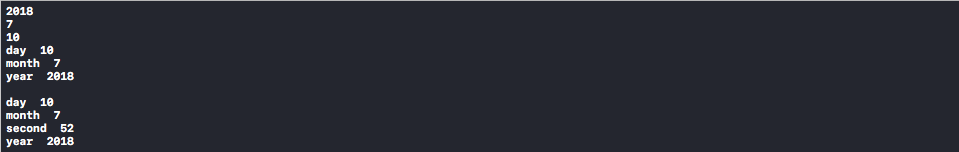if (m1.count("year")) {
cout << "year is in m1!" << endl;
} else {
cout << "year do not exist!" << endl;
}

for (map<string, int>::iterator it = m1.begin(); it != m1.end(); it++){
cout << it->first<<"  "<<it->second << endl;  //注意用法，不是用*it来访问了。first表示的是key，second存的是value.也就是在map中存储的又是另外一种数据结构（pair）
}

map<string, int> m_map = {{"key1", 1}, {"key2", 2}, {"key3", 3}, {"key4", 4}};
auto itr = m_map.find("key2");
while (itr == m_map.end()) {
(itr->second)++;
}

vector<int> ivec = {2, 1, 3, 2, 2, 2, 3, 4, 6, 7, 8, 9, 2};
set<int> set2;
map<string, int> m_map = {{"key1", 1}, {"key2", 2}, {"key3", 3}, {"key4", 4}};
auto itr               = m_map.find("key2");
while (itr == m_map.end()) {
(itr->second)++;
}
m_map.insert(pair<string, int>("key5", 100));
for (auto &x : m_map) {
if (x.first == "key3")
cout << "1 " << x.first << ":" << x.second << endl;
else
cout << "2 " << x.first << ":" << x.second << endl;
}
pair<string, int> authors = {"key6", 111};
pair<string, int> p("key7", 2222);
pair<string, int> p2("key8", 122);
m_map.insert({p2, authors, p});
m_map.insert(map<string, int>::value_type("key9", 1111));
auto ret = m_map.insert({"key9", 1111});
pair<map<string, int>::iterator, bool> ret2; //非泛型
if (!ret.second)
++ret.first->second;
auto map_it = m_map.cbegin();
while (map_it != m_map.cend()) {
cout << map_it->first << " key " << map_it->second << " value" << endl;
++map_it;
}
set2.insert(ivec.cbegin(), ivec.cend());
set2.insert({11, 22, 33, 44});
set2.insert(set<int>::value_type(11111));
auto set_it = set2.cbegin();
while (set_it != set2.end()) {
cout << *set_it << endl;
++set_it;
}

# multimap

multimap也是可以反转的，经过排序的关联容器，但键和值的类型不同，且同一个键可能与多个值相关联。

# 四种无关联容器

1.unordered_set

2.unordered_multiset

3.unordered_map

4.unordered_mutimap

11-27
03-1903-062万+
01-118万+
05-072822
07-221142
04-061905
05-29
03-06250
12-131万+
02-09157